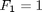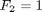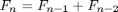## Loren on the Art of MATLABTurn ideas into MATLAB

Note

Loren on the Art of MATLAB has been archived and will not be updated.

# Fibonacci and filter

Over the years, people have posted many versions of code for calculating Fibonacci numbers. I thought I'd collect a few of the ideas here and start a discussion on the merits of the different versions.

### Fibonacci Numbers

Here's a place to get some history about the man and his work. Over the centuries, people have that these numbers seem to come up in the natural world. Try searching for Fibonacci and sunflower; I just got 31,600 hits. Fibonacci numbers are a sequence of numbers that can be derived via a recurrence relationship. Start the sequence with two |1|s. Then, each subsequent number in the sequence is found by adding the two most recent numbers. Mathematically stated:### Straightforward for-loop

Let's calculate a reasonable chunk of the sequence through the straightforward method suggested by the formula above.

clear
nf = 102;
tic
fibf(1) = 1;
fibf(2) = 1;
for n = 3:nf
fibf(n) = fibf(n-1)+fibf(n-2);
end
ftimes(1) = toc;  % Collect tic-toc times.

And now let's look at the first few numbers to be sure we're getting the expected answers.

fibf(1:10)
ans =
1     1     2     3     5     8    13    21    34    55


### for-loop with Preallocation

You've all heard it before. One reason the former calculation took so much time is at least alleged to be because of growing the array fibf each time through the loop. So now let's use the same loop, but preallocate the output array so it doesn't need to grow during the calculation. We'll compare all the computation times later on in this post.

tic
fibp = ones(1,nf);
for n = 3:nf
fibp(n) = fibp(n-1) + fibp(n-2);
end
ftimes(end+1) = toc;

### Recursive Function

The mathematical formula above suggests that we could write a Fibonacci sequence algorithm using a recursive function, i.e., one that calls itself. Here's what I came up with. Note that this version grows an array each time. I might have been able to be clever about this. I doubt the code would be as clear, however. Feel free to post a version to prove me wrong!

type fibrec
function f = fibrec(n)
%FIBREC Recursive function for n Fibonacci numbers.

% Minimize the error checking so we don't bog down in it. I have included it
% if ~isscalar(n) | ~isreal(n) | n<0 | fix(n)~=n
%    error('ArtBlog:fibrec:MBRealPosInt','N must be a real positive integer')
% end
if n == 1,
f = 1;   % First element is 1.
return;
elseif n == 2
f = [1 1];  % First two elements are 1.
else
% Call fibrec with previous result and compute next one from it.
fm1 = fibrec(n-1);
f = [fm1 fm1(end)+fm1(end-1)];
end


And now let's use it to calculate the first chunk of Fibonacci numbers.

tic
fibr = fibrec(nf);
ftimes(end+1) = toc;

### Use filter to Calculate the Sequence

I have one other idea now, to use the function filter. Looking at the help,

help filter
 FILTER One-dimensional digital filter.
Y = FILTER(B,A,X) filters the data in vector X with the
filter described by vectors A and B to create the filtered
data Y.  The filter is a "Direct Form II Transposed"
implementation of the standard difference equation:

a(1)*y(n) = b(1)*x(n) + b(2)*x(n-1) + ... + b(nb+1)*x(n-nb)
- a(2)*y(n-1) - ... - a(na+1)*y(n-na)

If a(1) is not equal to 1, FILTER normalizes the filter
coefficients by a(1).

FILTER always operates along the first non-singleton dimension,
namely dimension 1 for column vectors and non-trivial matrices,
and dimension 2 for row vectors.

conditions, Zi and Zf, of the delays.  Zi is a vector of length
MAX(LENGTH(A),LENGTH(B))-1, or an array with the leading dimension
of size MAX(LENGTH(A),LENGTH(B))-1 and with remaining dimensions
matching those of X.

FILTER(B,A,X,[],DIM) or FILTER(B,A,X,Zi,DIM) operates along the
dimension DIM.

Overloaded functions or methods (ones with the same name in other directories)
help timeseries/filter.m

Reference page in Help browser
doc filter



I see that it could be helpful since prior output values within the filtered array can be used to calculate subsequent ones. I want the latest one to add together the previous two outputs. My input, x is the array [1 1 0 0 ... 0]. And for each output, I need only consider the current input, assuming I pad my original starting value of 1 with zeros. Therefore, for this case, b = 1. The leading coefficient, multiplying the last output, is 1. And then I want to add the two previous outputs, but in the equation, these appear with -a(k), so the a portion of the filter is [1 -1 -1].

tic
x = [1 zeros(1,nf-1)];
a = [1 -1 -1];
b = 1;
fibfil = filter(b, a, x);
ftimes(end+1) = toc;

Let's be sure I got the right answer.

isequal(fibf, fibp, fibr, fibfil)
ans =
1


### isequal Tip

Did you know isequal could take more than two inputs? If you store your items to compare in a cell array, C, you can then do this: passfail = isequal(C{:}):, taking advantage of the comma-separated list notation.)

### Compare Computation Times

It's time to see how quickly the various methods performed. I have multiplied the times by 1000 to make reading the numbers easier.

fprintf('%s\n\n','Compare Times (in milliseconds)')
meths = ['loop           ',...
'prealloc       ',...
'recursive      ',...
'filter         '];
fprintf('%s\n\n',meths)
fprintf('%2.9f    ', ftimes*1000), fprintf('\n')
Compare Times (in milliseconds)

loop           prealloc       recursive      filter

0.329650836    0.243606380    2.244419333    0.058666674


### filter is Useful for Vectorization

I've shown one example here in which filter was useful for vectorizing code and make it perform well. Another one I know about is code from John D'Errico for calculating a moving window standard deviation. There are probably others. Do you know of other good uses for filter, besides straightforward applications for filtering and convolving signals? Let me know.

Published with MATLAB® 7.2